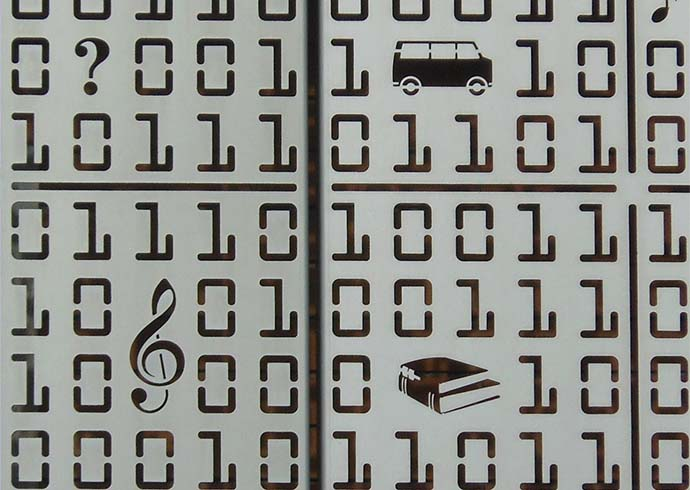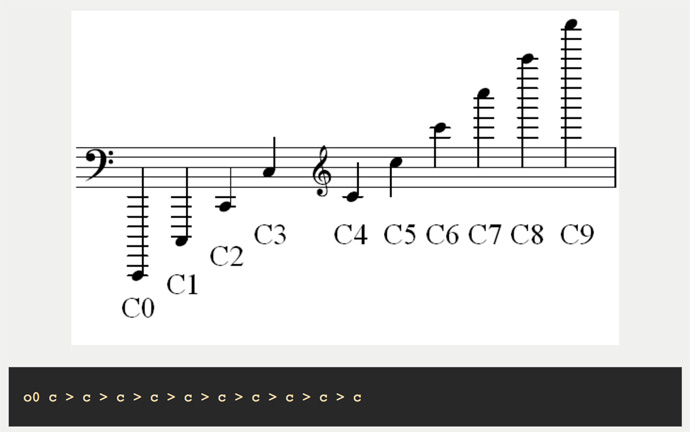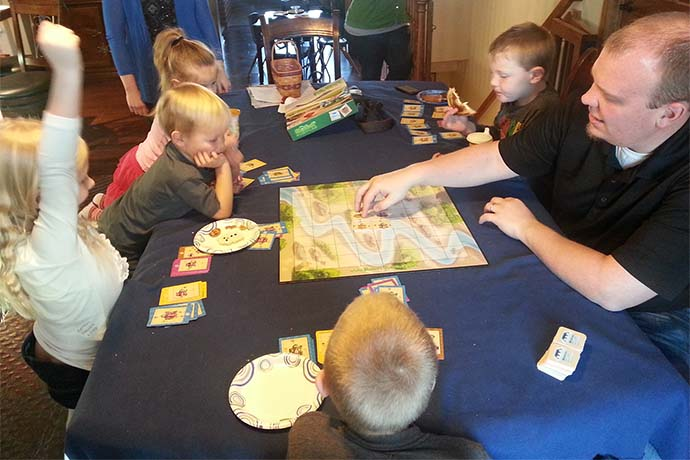## Searching for Prime NumbersFiguring out how to find prime numbers is a matter of the right strategy plus code plus trying different ideas.

### Numbers are fascinating

I’ve always been fascinated with numbers. Like the fact that when you add up the digits of any number which is divisible by 9, the sum adds up to either 9 or another number which is divisible by 9:

9 x 678 = 6102
6 + 1 + 0 + 2 = 9

### Prime numbers

One evening whilst working away in Scotland, my boss and I were talking about number theory and the subject of prime numbers came up. He told me there are prizes for finding new prime numbers that are hundreds of digits long. He explained to me that they use supercomputers to search for prime numbers. I hadn’t thought much about prime numbers before and I didn’t know about this search for them. It captured my imagination and set my mind off wondering how big a prime number I could find.

### The challenge

He bet me a bacon sandwich that I couldn’t find a 7 digit prime number by the time we met up again in the morning, without cheating. I thought how hard could it be and I accepted the challenge. Surely I could do this. I just needed to write a program and leave it running overnight. I was not going to lose this bet, there was a bacon sandwich riding on it.

### What is a prime number?

A prime number can be divided only by itself or by 1. For example, 101 is a prime number because 101 divided by 101 equals 1 and 101 divided by 1 equals 101. The number 101 divided by any other number results in a non-whole number with a remainder. The number 9 is not a prime number because it can be divided by itself (9), by 1, and by 3.

Prime numbers are whole numbers greater than 1. Apart from number 2, prime numbers are always odd numbers, because all even numbers can always divide by 2 as well as themselves and 1.

### Getting ready for the challenge

I went to the garage over the road to get some supplies for the night ahead and went back to my hotel room to start the search for prime numbers.

I started out by writing the basic logic of what was required, onto paper:

• 2 is the first prime number, but it is even. Start the search from 3 and add 2 each time to it so I am only ever checking odd numbers.
• Check the number is not divisible by any of the prime numbers lower than it.
• Print the number to the screen.
• Keep a record of all of the prime numbers I find so I can leave it running and show my boss the next morning.

### Turning this first attempt into code

The below code snippet shows how I was checking for prime numbers. I have limited the search to be between a start number and end number as it is just an example.

```using System;
using System.Collections.Generic;
using System.Linq;

{
public static class PrimeCheck
{

public static void Main()
{
}

public static void SearchForPrimeNumbers(long lowestNumberToCheck, long highestNumberToCheck)
{
const long LOWEST_PRIME_NUMBER = 2;
List<long> foundPrimes = new List<long>();

long numberToCheck = lowestNumberToCheck;

Console.WriteLine("Searching for prime numbers between {0} and {1}", lowestNumberToCheck, highestNumberToCheck);

while (numberToCheck <= highestNumberToCheck)
{
{
Console.WriteLine("Prime number: " + numberToCheck.ToString());
}
numberToCheck = numberToCheck + 2;
}

Console.WriteLine("Longest Prime: {0} - {1} digits", foundPrimes.Last(), foundPrimes.Last().ToString().Length);
}

private static bool IsPrimeNumber(List<long> foundPrimes, long numberToCheck)
{
bool isPrime = true;
foreach (long foundPrime in foundPrimes)
{
if (numberToCheck % foundPrime == 0)
{
isPrime = false;
break;
}
}
return isPrime;
}

}
}
```

### How this code works

The `using System`, `using System.Collections.Generic`, and `using System.Linq` (lines 1-3) tells our code to load in these common libraries of code classes, objects, methods, and other code so we can use their code without having to write new code.

The `namespace PrimeNumbers` on line 5 tells the programming language, .Net in this case, that all of the code is a single logical group, called a namespace, and referenced with the name `PrimeNumbers`. Namespaces help prevent conflicts between sections of code.

Once we load the common libraries and set the `namespace PrimeNumbers`, next we create a class called `PrimeCheck` on line 7. This class holds objects, methods, definitions, and other code we’ll use to calculate numbers as we search for prime numbers.

Within the `PrimeCheck` class, we have three methods, or blocks of code, to search for prime numbers:

1. Our first and primary method is called `Main()` (lines 10-13) which is called first when we run our code. Within `Main()`, we call the `SearchForPrimeNumbers` method and pass two parameters, `3` and `1000`. We’ll explain these parameters in a moment.
2. Next, we define the second method called `SeachForPrimeNumbers` on lines 15-37. It includes two values that must be passed into it, a value called `lowestNumberToCheck` and another value called `highestNumberToCheck`. If you remember the discussion above, we want to start with the number `3` because it is the first non-even number and, therefore, might be a prime number. We set the `highestNumberToCheck` value to `1000` but it could be any number. If 1000 is the largest number we check, then we will get one, two, and three digit prime numbers.
3. Within the `SearchForPrimeNumbers` method, we run a series of tests on our numbers to test and identify prime numbers between our lowest start number (`3`) and highest end number (`1000`). First, a constant `LOWEST_PRIME_NUMBER` is set equal to `2` (line 17). Then we create a list variable called `foundPrimes` (line 18) to hold the prime numbers we find and start by adding the `LOWEST_PRIME_NUMBER` value of `2` to our list variable on line 19.
4. We also set a long integer (number) variable called `numberToCheck` equal to the `lowestNumberToCheck` parameter we passed into this method (line 21). In our case, if you remember, we passed in the integer `3` the `SearchForPrimeNumbers` method with our `Main()` method.
5. We also set another long integer variable called `latestPrimeNumber` and set it equal to the first item in our `foundPrimes` list variable on line 22.
6. The `SearchForPrimeNumbers` method creates a list of prime numbers found, if any, and prints the numbers to the computer screen with the `Console.WriteLine` method on lines 24 and 31. The `{0}` and `{1}` are replaced by the values of the `lowestNumberToCheck` and `highestNumberToCheck` variables, respectively.
7. In addition to the `SearchForPrimeNumbers` method, we also set a third method called `IsPrimeNumber` on lines 39-51. This method is called within the `SearchForPrimeNumber` method (lines 28-32) to confirm whether or not a number is prime. The `IsPrimeNumber` method accepts two values, one is a list of prime numbers `foundPrimes` found with our code and the other value is a number to check `numberToCheck` if it is prime.
8. Within the `IsPrimeNumber` method, we set a boolean variable `isPrime` equal to `true` on line 41.
9. Next, we use a foreach statement to determine if numbers in the `foundPrimes` list passed into this method are prime numbers (or not). If the `numberToCheck` can be divided by one of the prime numbers in our `foundPrimes` list, then the `isPrime` variable is set to `false` on line 46.
10. If the `isPrimeNumber` method returns `true`, the `SearchForPrimeNumbers` method uses `Console.WriteLine` on line 36 to print to the computer screen the value of `numberToCheck` and the length of this variable, the number of its digits.

### Results of the challenge

I left the code running for 5 or 6 hours and when I checked it in the morning, I was amazed to to see that not only had I found 7 digit prime numbers, I had also found 8 digit ones.

My boss was very surprised and at first he thought I must have cheated. I showed him the massive table of prime numbers and he conceded that I had won the challenge. I got my bacon sandwich!

Later that day, on the way to the airport, I realised where I could fine-tune the application to eliminate checks against unnecessary number and make it run even faster.

### Improving the algorithm

I was checking if a number was divisible by every prime number lower than it, which was wasting a lot of time.

All I needed to do was test the number against prime numbers which were less than or equal to the square root of the number.

Take the prime number 31, for example, divided by these prime number factors:

Prime Factor Result
31 / 3 = 10.34
31 / 5 = 6.2
square root of 31 = 5.57
31 / 7 = 4.43
31 / 11 = 2.82
31 / 13 = 2.38
31 / 17 = 1.82
31 / 19 = 1.63
31 / 23 = 1.35
31 / 29 = 1.07

Look at the factor numbers in the middle column and the results in the right hand column, for example, the prime number factor of 3 and 10.34 result in the first calculation.

To start with, the factor prime number in the middle is lower than the result number on the right.

As the factor number in the middle gets higher than the square root of 31 (which is 5.57), you will notice the result number on the right gets lower than the factor prime number in the middle. We already know it doesn’t divide by the numbers lower than the square root as we have already tested 3 and 5.

In this example I was able to find out that the number was prime by just doing 2 checks rather than 9.

We altered the code and it sped through the prime numbers far faster than the previous code. We left it running for a few days and managed to get to a 20 digit prime number.

This might not be very impressive to you, but we were doing this from my personal laptop, which by today’s standard’s is not very powerful.

Here is the improved code that uses the square root of a number to reduce the number of checks to see if it is a prime number:

```using System;
using System.Collections.Generic;
using System.Linq;

{
public static class PrimeCheck
{

public static void Main()
{
}

public static void SearchForPrimeNumbers(long lowestNumberToCheck, long highestNumberToCheck)
{
const long LOWEST_PRIME_NUMBER = 2;
List<long> foundPrimes = new List<long>();

long numberToCheck = lowestNumberToCheck;

Console.WriteLine("Searching for prime numbers between {0} and {1}", lowestNumberToCheck, highestNumberToCheck);

while (numberToCheck <= highestNumberToCheck)
{
long maxPrime = (long)Math.Ceiling(Math.Sqrt(numberToCheck));
{
Console.WriteLine("Prime number: " + numberToCheck.ToString());
}
numberToCheck = numberToCheck + 2;
}

Console.WriteLine("Longest Prime: {0} - {1} digits", foundPrimes.Last(), foundPrimes.Last().ToString().Length);
}

private static bool IsPrimeNumber(List<long> foundPrimes, long numberToCheck, long maxPrime)
{
bool isPrime = true;
foreach (long foundPrime in foundPrimes)
{
if(foundPrime > maxPrime)
{
isPrime = true;
break;
}
else
{
if (numberToCheck % foundPrime == 0)
{
isPrime = false;
break;
}
}
}
return isPrime;
}

}
}
```

You can test this code in your browser on dotnetfiddle here.

Here’s how the new code works:

1. First, we define a long integer (number) variable called `maxPrime`, on line 28, and set it equal to calculation of the square root of the `numberToCheck` variable we defined. Then we pass our new `maxPrime` variable as a third parameter to the `IsPrimeNumber` method to check if it is a prime number, on line 29.
2. Next, we update the `IsPrimeNumber` method to accept a third value, a long integer called `maxPrime` on line 40.
3. Within our foreach loop in the `IsPrimeNumber` method, we add a condition (on lines 45-51) to evaluate whether or not the maxPrime parameter value passed in is greater than each prime number listed in our foundPrimes list. If greater than, the `isPrime` variable is set to `true`.

You can test this code in your browser on dotnetfiddle. See below for the link.

In my actual program, I got it to store every prime number in a database table, so I was able to stop the search and continue it at a later date.

Why don’t you have a play and see what the longest prime number you can find is? Use your computer or dotnetfiddle or LINQPad, a free editing tool to test code.

There is another improvement to be made to the code in terms of the maxPrime value. I wonder if you can work it out. Calculating the square root of each possible prime number uses a lot of computer resources. However, there is an interesting relationship between any number and its square. The number 2, for example, is 50% of its square of 4 while 3 is 33% of its square of 9, 4 is 25% of its square of 16, and 5 is 20% of its square of 25. Could you use this decreasing percentage ratio instead of calculating square roots to determine if a number is prime?

#### Searching for Prime Numbers

http://www.codeshare.co.uk/blog/searching-for-prime-numbers/

## Author

•Paul Seal is a .NET Web Developer from Derby (UK) who specialises in building Content Management System (CMS) websites using MVC with Umbraco as a framework. Paul is passionate about web development and programming as a whole. Apart from when he's with my wife and son, if he's not writing code, he's thinking about it or listening to a podcast about it.

### Also In The June 2016 IssueTinkercad makes it easy to create and print 3D objects from your designs and designs others create.Bakuro puzzles are a fun way to learn about binary numbers and how to calculate their values.Figuring out how to find prime numbers is a matter of the right strategy plus code plus trying different ideas.There are many ways to learn technology while playing. Here are technologies and resources you might want to find online this summer.A super portable version of Makey Makey, there's lots of experiments you can do with the new Makey Makey Go!A computational fairytale updated for the computer age, a grasshopper learns algorithms and planning ahead.A book about the daily life of many different programmers who do neat things with code.The US Congressional App Challenge is an annual contest to encourage US high school students to try programming by creating an app.The Alda programming language lets you write and play music on your computer by typing simple commands.Younger kids can have lots of fun playing games this summer while learning basic programming concepts.Minecraft is a fun game to explore with a vast set of worlds, animals, and adventures. Here are ideas to continue the adventure, online and offline.Battery history is a critical part of the history of technology. Without stored electricity, there would be no electronics.Links from the bottom of all the June 2016 articles, collected in one place for you to print, share, or bookmark.Interesting stories about computer science, software programming, and technology for June 2016.A Swiss-made robot, Thymio robots work with drag and drop languages and text-based languages like JavaScript.Interested but not ready to subscribe? Sign-up for our free monthly email newsletter with curated site content and a new issue email announcement that we send every two months.

No, thanks!